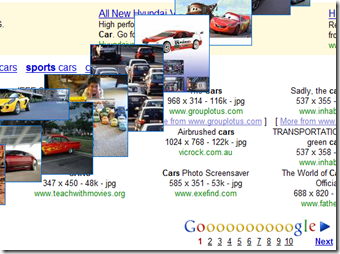# Let the Google Image Search Dance

Want to see the thumbnail images in Google Image Search to dance on a Chinese Song. Just try this cool trick to make them do so.1. Search for some images at Google Image Search.
3.  `javascript:R= 0; x1=.1; y1=.05; x2=.25; y2=.24; x3=1.6; y3=.24; x4=300; y4=200; x5=300; y5=200; DI= document.images ; DIL=DI.length; function A(){for(i=0; i
1.Anonymous